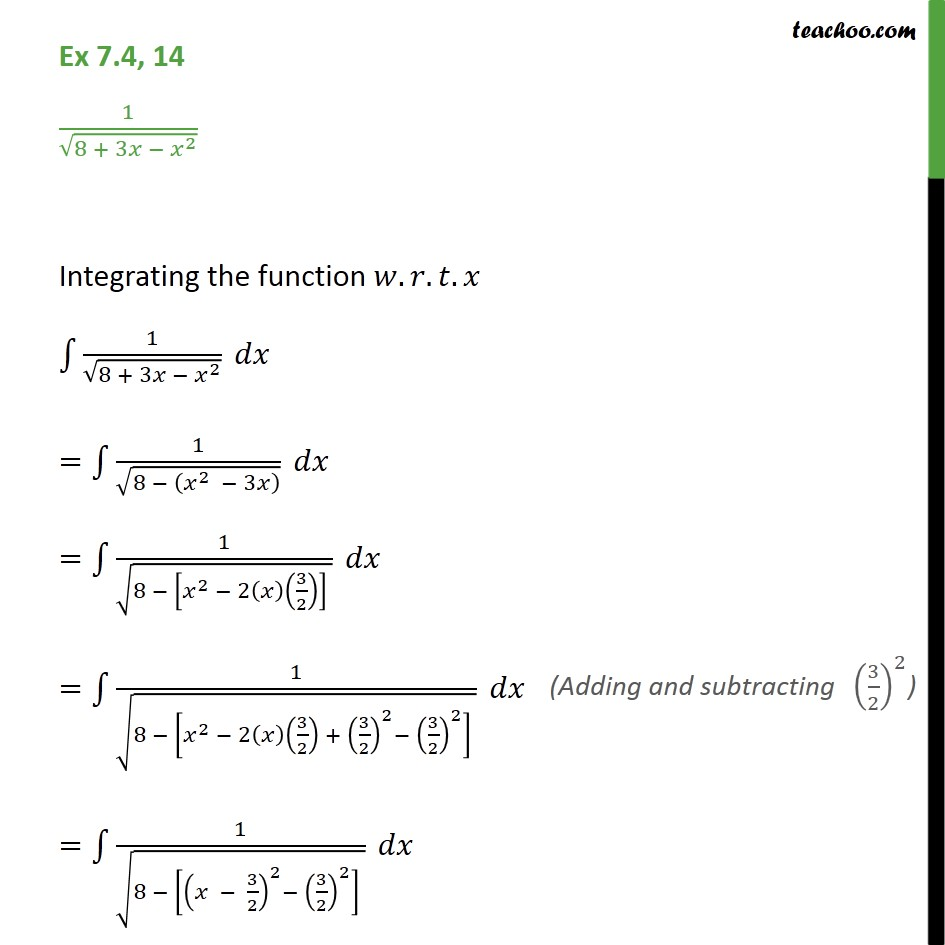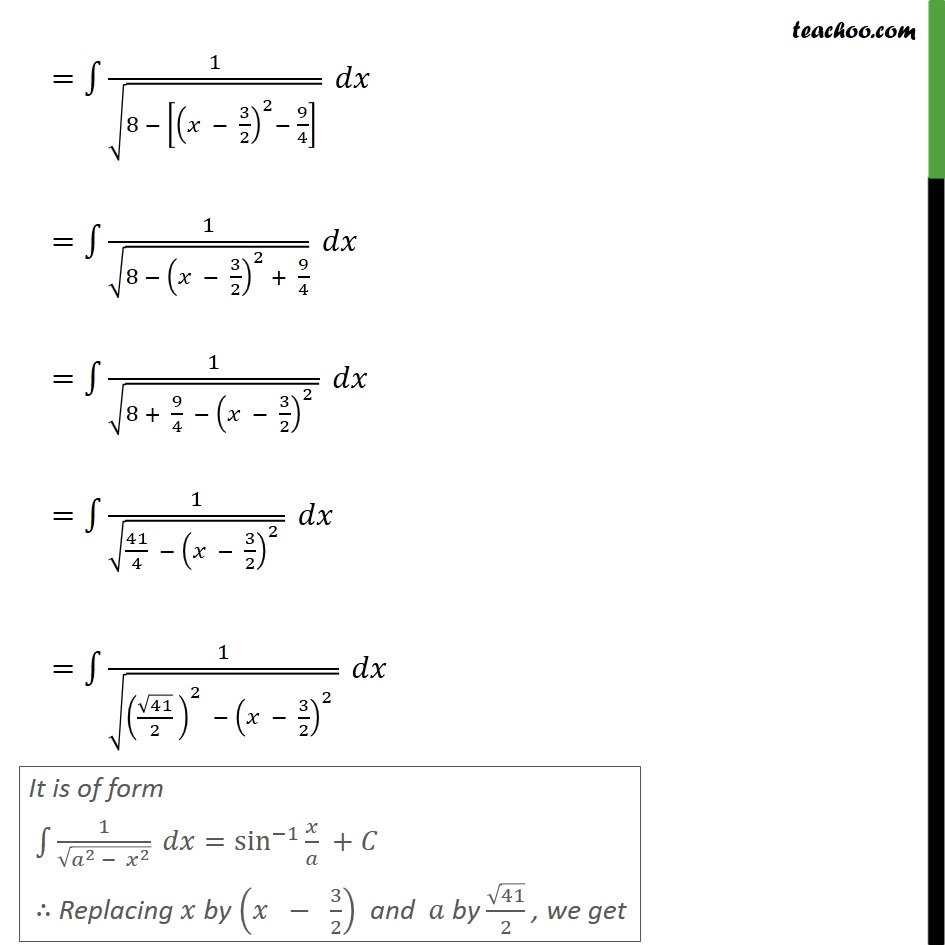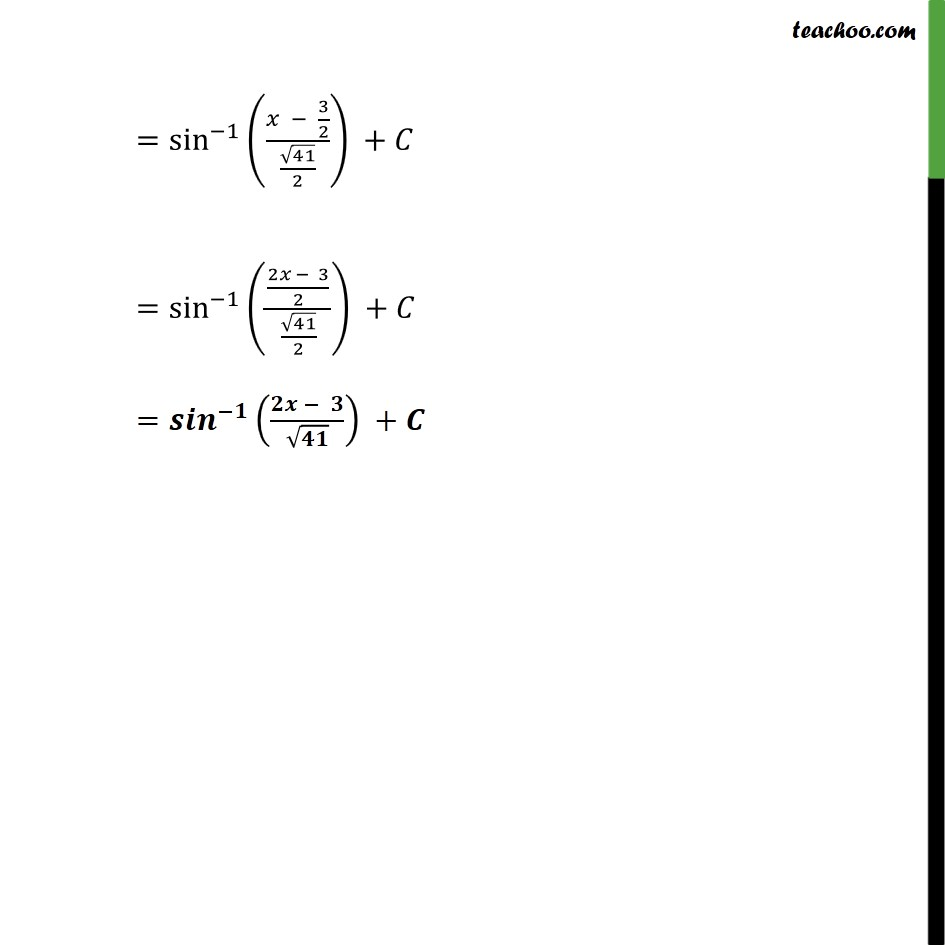Ex 7.4

Chapter 7 Class 12 Integrals
Serial order wiseLearn in your speed, with individual attention - Teachoo Maths 1-on-1 Class

### Transcript

Ex 7.4, 14 1 8 + 3 2 Integrating the function . . . 1 8 + 3 2 = 1 8 2 3 = 1 8 2 2 3 2 = 1 8 2 2 3 2 + 3 2 2 3 2 2 = 1 8 3 2 2 3 2 2 = 1 8 3 2 2 9 4 = 1 8 3 2 2 + 9 4 = 1 8 + 9 4 3 2 2 = 1 41 4 3 2 2 = 1 41 2 2 3 2 2 = sin 1 3 2 41 2 + = sin 1 2 3 2 41 2 + = +